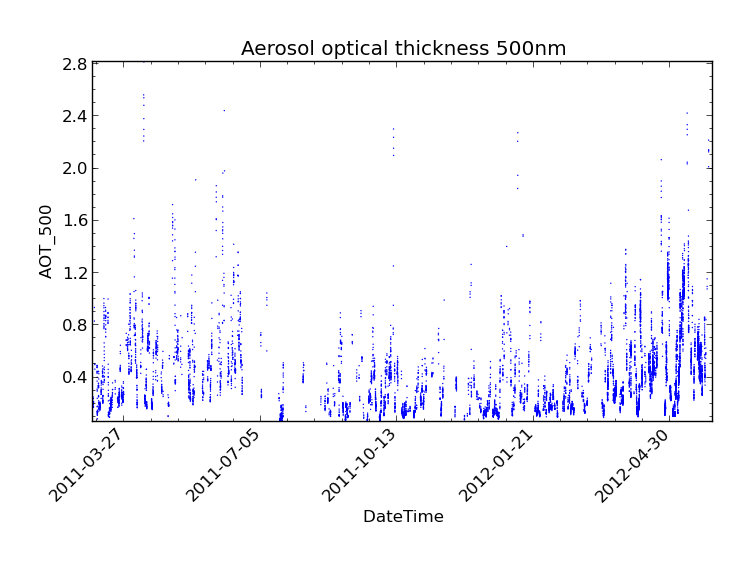# 12. Statistics¶

The Community Intercomparison Suite allows you to perform statistical analysis on two variables using the ‘stats’ command. For example, you might wish to examine the correlation between a model data variable and actual measurements. The ‘stats’ command will calculate:

1. Number of data points used in the analysis.
2. The mean and standard deviation of each dataset (separately).
3. The mean and standard deviation of the absolute difference (var2 - var1).
4. The mean and standard deviation of the relative difference ((var2 - var1) / var1).
5. The Linear Pearson correlation coefficient.
6. The Spearman Rank correlation coefficient.
7. The coefficients of linear regression (i.e. var2 = a var1 + b ), r-value, and standard error of the estimate.

These values will be displayed on screen and can optionally be save as NetCDF output.

Note

Both variables used in a statistical analysis must be of the same shape in order to be compatible, i.e. the same number of points in each dimension, and of the same type (ungridded or gridded). This means that, for example, operations between different data products are unlikely to work correctly - performing a collocation or aggregation onto a common grid would be a good pre-processing step.

Note

Only points which have non-missing values for both variables will be included in the analysis. The number of points this includes is part of the output of the stats command.

Warning

Unlike aggregation, `stats` does not currently use latitude weighting to account for the relative areas of different grid cells.

The statistics syntax looks like this:

```\$ cis stats <datagroup>... [-o <outputfile>]
```

where:

`<datagroup>`

is a CIS datagroup specifying the variables and files to read and is of the format `<variable>...:<filename>[:product=<productname>]` where:

• `<variable>` is a mandatory variable or list of variables to use.
• `<filenames>` is a mandatory file or list of files to read from.
• `<productname>` is an optional CIS data product to use (see Data Products):

One or more datagroups should be given, but the total number of variables declared in all datagroups must be exactly two. See Datagroups for a more detailed explanation of datagroups.

`<outputfile>`
is an optional argument specifying a file to output to. This will be automatically given a `.nc` extension if not present. This must not be the same file path as any of the input files. If not provided, then the output will not be saved to a file and will only be displayed on screen.

## 12.1. Statistics Example¶

In this example, we perform a statistical comparison of Aeronet aerosol optical thickness at two wavelengths. The data we are using is shown in the following CIS plot commands and can be found at `/group_workspaces/jasmin/cis/data`:

```\$ cis plot AOT_500:aeronet/AOT/LEV20/ALL_POINTS/920801_121229_Yonsei_University.lev20 --title "Aerosol optical thickness 550nm"
\$ cis plot AOT_440:aeronet/AOT/LEV20/ALL_POINTS/920801_121229_Yonsei_University.lev20 --title "Aerosol optical thickness 440nm"
```We then perform a statistical comparison of these variables using:

```\$ cis stats AOT_500,AOT_440:aeronet/AOT/LEV20/ALL_POINTS/920801_121229_Yonsei_University.lev20
```

Which gives the following output:

```===================================================================
RESULTS OF STATISTICAL COMPARISON:
-------------------------------------------------------------------
Compared all points which have non-missing values in both variables
===================================================================
Number of points: 10727
Mean value of dataset 1: 0.427751965508
Mean value of dataset 2: 0.501316673814
Standard deviation for dataset 1: 0.307680514916
Standard deviation for dataset 2: 0.346274598431
Mean of absolute difference: 0.0735647083061
Standard deviation of absolute difference: 0.0455684788406
Mean of relative difference: 0.188097066086
Standard deviation of relative difference: 0.0528621773819
Spearman's rank coefficient: 0.998289763952